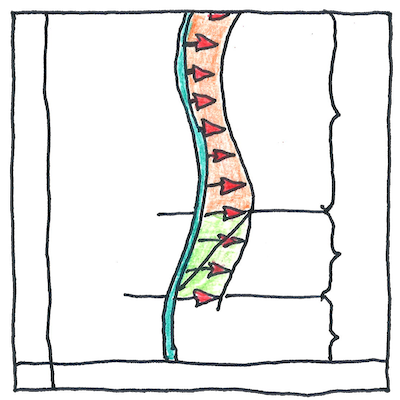# 1918

## The book of science

Tom Sharp

 Emmy Noether physics

## Noether’s theorem

• Every physical continuous symmetry
• has a corresponding conservation law.
• The principle of least action
• and the translational symmetry of space
• result in Newton’s first law of motion,
• that is, the conservation of linear momentum.
• The conservation of energy,
• that is, the first law of thermodynamics,
• results from the translational symmetry of time
• and the principle of least action.
• Emmy Noether proved this correspondence
• exists for all differentiable symmetries.

## Spherical symmetry

• Turn a perfect sphere
• of uniform color and texture
• in any direction on any axis
• at any time in any place
• and you’ll never notice
• whether anything is turning.

## Sense of things

• Noether’s theorem suggests
• that things make sense,
• or would make sense
• if we had a way to comprehend them.
• It might be a matter of faith
• as to whether our universe
• is necessary or random,
• but at least it’s internally consistent.

With respect to physical laws, one point in space is no different from any other. This kind of symmetry and the idea that the universe, like an undisturbed bubble of water suspended weightless in space, assumes the state of least energy, a perfect sphere, results in the conservation of momentum. Similarly, gauge symmetry results in conservation of electric charge, and rotation symmetry results in conservation of angular momentum.